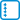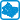## Linear recurrences

Find the formula (the analytical expression) for the $$n$$-th element of the sequence specified by the following recurrences:

• #### Variant

$$a_0=1, a_{n+1}=a_n+1$$

• #### Variant

$$a_0=1, a_{n+1}=2a_n+3$$

• #### Variant

$$a_0=a_1=1, a_{n+2}=a_{n+1}+6a_n$$

• #### Variant

$$a_0=a_1=1, a_{n+2}=a_{n+1}+6a_n-4$$

• #### Variant

$$a_0=0, a_1=1, a_{n+2}=4(a_{n+1}-a_n)$$

• #### Variant

$$a_0=0, a_1=1, a_{n+2}=4(a_{n+1}-a_n)+1$$

• #### Variant

$$a_0=2, a_1=3, a_{n+2}=3a_n-2a_{n+1}$$

• #### Variant

$$a_0=0, a_1=1, a_{n+2}=a_{n+1}+2a_n+2$$

• #### Variant

$$a_0=a_1=1, 5a_{n+2}=4a_{n+1}-a_n$$

• #### Variant

$$a_0 = 4, a_1 = 3, a_n = a_{n-1} + 2 a_{n-2} + 3{\cdot} 2^n$$ for $$n \geq 2$$